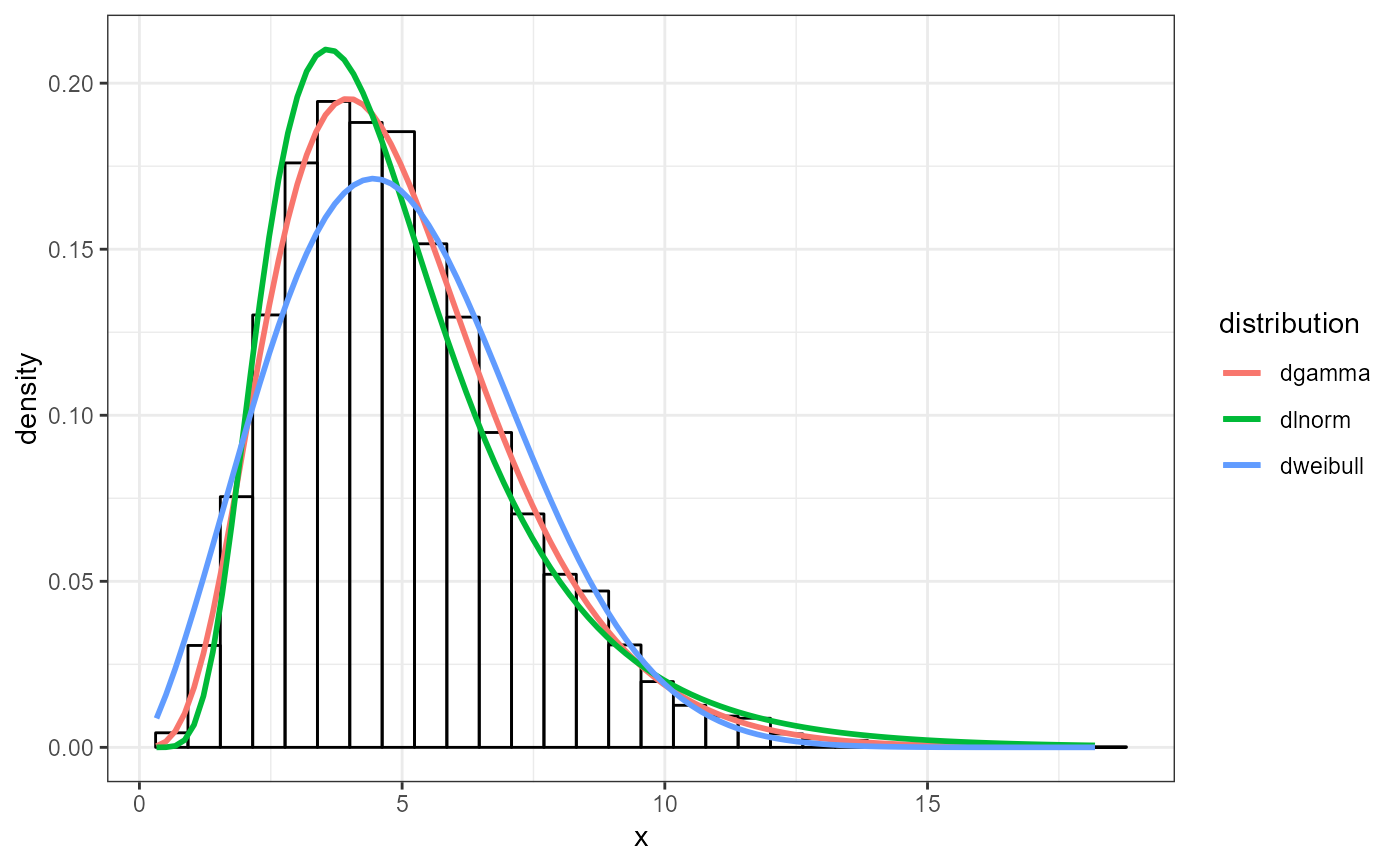Density Comparison Plot

plot_density(x, fits, nbins)

## Arguments

x numeric vector of sample data a list object produced from fit_univariate, fit_empirical, or fit_univariate_man number of bins for histogram

## Value

ggplot of empirical histogram of x compared to theoretical density distributions

## Examples

library(ggplot2)#> Warning: package 'ggplot2' was built under R version 3.4.4set.seed(37)
x <- rgamma(10000, 5)
dists <- c('gamma', 'lnorm', 'weibull')
fits <- lapply(dists, fit_univariate, x = x)
plot_density(x, fits, 30) +
theme_bw()